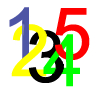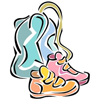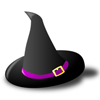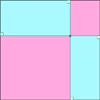# Resources tagged with: 2D shapes and their properties

Filter by: Content type:
Age range:
Challenge level:

### There are 61 results

Broad Topics > Angles, Polygons, and Geometrical Proof > 2D shapes and their properties### A Rational Search

##### Age 16 to 18Challenge Level

Investigate constructible images which contain rational areas.### Spirostars

##### Age 16 to 18Challenge Level

A spiropath is a sequence of connected line segments end to end taking different directions. The same spiropath is iterated. When does it cycle and when does it go on indefinitely?### Spokes

##### Age 16 to 18Challenge Level

Draw three equal line segments in a unit circle to divide the circle into four parts of equal area.### Track Design

##### Age 14 to 16Challenge Level

Where should runners start the 200m race so that they have all run the same distance by the finish?### Ball Bearings

##### Age 16 to 18Challenge Level

If a is the radius of the axle, b the radius of each ball-bearing, and c the radius of the hub, why does the number of ball bearings n determine the ratio c/a? Find a formula for c/a in terms of n.### Gym Bag

##### Age 11 to 16Challenge Level

Can Jo make a gym bag for her trainers from the piece of fabric she has?### Witch's Hat

##### Age 11 to 16Challenge Level

What shapes should Elly cut out to make a witch's hat? How can she make a taller hat?### Efficient Packing

##### Age 14 to 16Challenge Level

How efficiently can you pack together disks?### Pentagonal

##### Age 14 to 16Challenge Level

Can you prove that the sum of the distances of any point inside a square from its sides is always equal (half the perimeter)? Can you prove it to be true for a rectangle or a hexagon?### Circles in Circles

##### Age 16 to 18Challenge Level

This pattern of six circles contains three unit circles. Work out the radii of the other three circles and the relationship between them.### Lunar Angles

##### Age 16 to 18Challenge Level

What is the sum of the angles of a triangle whose sides are circular arcs on a flat surface? What if the triangle is on the surface of a sphere?### Gold Yet Again

##### Age 16 to 18Challenge Level

Nick Lord says "This problem encapsulates for me the best features of the NRICH collection."### 2D-3D

##### Age 16 to 18Challenge Level

Two circles of equal size intersect and the centre of each circle is on the circumference of the other. What is the area of the intersection? Now imagine that the diagram represents two spheres of. . . .### Retracircles

##### Age 16 to 18Challenge Level

Four circles all touch each other and a circumscribing circle. Find the ratios of the radii and prove that joining 3 centres gives a 3-4-5 triangle.### Just Touching

##### Age 16 to 18Challenge Level

Three semi-circles have a common diameter, each touches the other two and two lie inside the biggest one. What is the radius of the circle that touches all three semi-circles?### Area I'n It

##### Age 16 to 18Challenge Level

Triangle ABC has altitudes h1, h2 and h3. The radius of the inscribed circle is r, while the radii of the escribed circles are r1, r2 and r3 respectively. Prove: 1/r = 1/h1 + 1/h2 + 1/h3 = 1/r1 +. . . .### Orthogonal Circle

##### Age 16 to 18Challenge Level

Given any three non intersecting circles in the plane find another circle or straight line which cuts all three circles orthogonally.### Dividing the Field

##### Age 14 to 16Challenge Level

A farmer has a field which is the shape of a trapezium as illustrated below. To increase his profits he wishes to grow two different crops. To do this he would like to divide the field into two. . . .### First Forward Into Logo 4: Circles

##### Age 7 to 16Challenge Level

Learn how to draw circles using Logo. Wait a minute! Are they really circles? If not what are they?##### Age 14 to 16Challenge Level

The sides of a triangle are 25, 39 and 40 units of length. Find the diameter of the circumscribed circle.### Semi-detached

##### Age 14 to 16Challenge Level

A square of area 40 square cms is inscribed in a semicircle. Find the area of the square that could be inscribed in a circle of the same radius.### Lawnmower

##### Age 14 to 16Challenge Level

A kite shaped lawn consists of an equilateral triangle ABC of side 130 feet and an isosceles triangle BCD in which BD and CD are of length 169 feet. A gardener has a motor mower which cuts strips of. . . .### Arclets Explained

##### Age 11 to 16

This article gives an wonderful insight into students working on the Arclets problem that first appeared in the Sept 2002 edition of the NRICH website.### Salinon

##### Age 14 to 16Challenge Level

This shape comprises four semi-circles. What is the relationship between the area of the shaded region and the area of the circle on AB as diameter?### Circumspection

##### Age 14 to 16Challenge Level

M is any point on the line AB. Squares of side length AM and MB are constructed and their circumcircles intersect at P (and M). Prove that the lines AD and BE produced pass through P.### LOGO Challenge 12 - Concentric Circles

##### Age 11 to 16Challenge Level

Can you reproduce the design comprising a series of concentric circles? Test your understanding of the realtionship betwwn the circumference and diameter of a circle.### LOGO Challenge - Circles as Animals

##### Age 11 to 16Challenge Level

See if you can anticipate successive 'generations' of the two animals shown here.### Approximating Pi

##### Age 14 to 18Challenge Level

By inscribing a circle in a square and then a square in a circle find an approximation to pi. By using a hexagon, can you improve on the approximation?### LOGO Challenge 11 - More on Circles

##### Age 11 to 16Challenge Level

Thinking of circles as polygons with an infinite number of sides - but how does this help us with our understanding of the circumference of circle as pi x d? This challenge investigates. . . .### LOGO Challenge 6 - Triangles and Stars

##### Age 11 to 16Challenge Level

Recreating the designs in this challenge requires you to break a problem down into manageable chunks and use the relationships between triangles and hexagons. An exercise in detail and elegance.### From All Corners

##### Age 14 to 16Challenge Level

Straight lines are drawn from each corner of a square to the mid points of the opposite sides. Express the area of the octagon that is formed at the centre as a fraction of the area of the square.### Trapezium Four

##### Age 14 to 16Challenge Level

The diagonals of a trapezium divide it into four parts. Can you create a trapezium where three of those parts are equal in area?### Curvy Areas

##### Age 14 to 16Challenge Level

Have a go at creating these images based on circles. What do you notice about the areas of the different sections?### Logosquares

##### Age 16 to 18Challenge Level

Ten squares form regular rings either with adjacent or opposite vertices touching. Calculate the inner and outer radii of the rings that surround the squares.### Kissing

##### Age 16 to 18Challenge Level

Two perpendicular lines are tangential to two identical circles that touch. What is the largest circle that can be placed in between the two lines and the two circles and how would you construct it?### Towering Trapeziums

##### Age 14 to 16Challenge Level

Can you find the areas of the trapezia in this sequence?### Squaring the Circle and Circling the Square

##### Age 14 to 16Challenge Level

If you continue the pattern, can you predict what each of the following areas will be? Try to explain your prediction.##### Age 14 to 16Challenge Level

Given a square ABCD of sides 10 cm, and using the corners as centres, construct four quadrants with radius 10 cm each inside the square. The four arcs intersect at P, Q, R and S. Find the. . . .### Floored

##### Age 14 to 16Challenge Level

A floor is covered by a tessellation of equilateral triangles, each having three equal arcs inside it. What proportion of the area of the tessellation is shaded?### LOGO Challenge 2 - Diamonds Are Forever

##### Age 7 to 16Challenge Level

The challenge is to produce elegant solutions. Elegance here implies simplicity. The focus is on rhombi, in particular those formed by jointing two equilateral triangles along an edge.### Tied Up

##### Age 14 to 16 ShortChallenge Level

How much of the field can the animals graze?### Three Four Five

##### Age 14 to 16Challenge Level

Two semi-circles (each of radius 1/2) touch each other, and a semi-circle of radius 1 touches both of them. Find the radius of the circle which touches all three semi-circles.### Holly

##### Age 14 to 16Challenge Level

The ten arcs forming the edges of the "holly leaf" are all arcs of circles of radius 1 cm. Find the length of the perimeter of the holly leaf and the area of its surface.##### Age 14 to 16Challenge Level

Investigate the properties of quadrilaterals which can be drawn with a circle just touching each side and another circle just touching each vertex.### Rhombus in Rectangle

##### Age 14 to 16Challenge Level

Take any rectangle ABCD such that AB > BC. The point P is on AB and Q is on CD. Show that there is exactly one position of P and Q such that APCQ is a rhombus.### Tricircle

##### Age 14 to 16Challenge Level

The centre of the larger circle is at the midpoint of one side of an equilateral triangle and the circle touches the other two sides of the triangle. A smaller circle touches the larger circle and. . . .### Pent

##### Age 14 to 18Challenge Level

The diagram shows a regular pentagon with sides of unit length. Find all the angles in the diagram. Prove that the quadrilateral shown in red is a rhombus.### Crescents and Triangles

##### Age 14 to 16Challenge Level

Can you find a relationship between the area of the crescents and the area of the triangle?### Semi-square

##### Age 14 to 16Challenge Level

What is the ratio of the area of a square inscribed in a semicircle to the area of the square inscribed in the entire circle?### LOGO Challenge 10 - Circles

##### Age 11 to 16Challenge Level

In LOGO circles can be described in terms of polygons with an infinite (in this case large number) of sides - investigate this definition further.